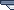New Home Please visit us at our new home. All content has been moved there.

## What is a Kilowatt Hour

The power company charges you for power in kilowatt hours, abbreviated kWh. This guide explains what a kilowatt hour is.

We all know that c are rated in watts, such as a 60 watt light bulb or a 100 watt light bulb. How much you pay for using a light bulb on depends on how long you leave it on for and the wattage rating of the light bulb.

Most power calculation is based on hours. If you turned a 100 Watt light bulb on for 1 hour, then you have used 100 Watt hours. Likewise if you turn the same light bulb on for 2 hours, then you have used 200 Watt hours. Notice that we simply multiply the watts of the light bulb by the number of hours it is left on.

Watt Hours = Watts Used * Hours Used

 Item used Time used for Watt hours Used 100 watt light bulb 1 hour 100 100 watt light bulb 5 hours 500 100 watt light bulb 24 hours 2,400 100 watt light bulb 24 hours a day for 30 days 72,000

The values in the last column get big fast when you start talking about how much power is used in a month. That is why most power use is measured in kilowatt hours. This simply means "1,000's of Watt hours". Using kilowatt hours, the above table becomes this.

kilowatt Hours Used = (Watts Used * Hours Used) / 1000

 Item used Time used for kilowatt hours Used 100 watt light bulb 1 hour 0.1 100 watt light bulb 5 hours 0.5 100 watt light bulb 24 hours 2.4 100 watt light bulb 24 hours a day for 30 days 72

This last equation is the one that you should remember when doing kilowatt hour calculations.

kilowatt Hours = (Watts Used * Hours Used) / 1000

If you want to know how much you pay for a kilowatt hour, then you should check our our guides to help you Determine How Much You Pay For Electricity.

Lets do some examples.

Example 1: If you used a 1000 watt curling iron for 1 hour, how many kilowatt hours have you used?

kilowatt Hours = (1000 watts * 1 hour) / 1000 = 1 kWh

Example 2: If you used a 10,000 watt oven for 3 hours, how many kilowatt hours have you used?

kilowatt Hours = (10,000 watts * 3 hours) / 1000 = 30 kWh

We also have a guide to help you learn to Read You Power Meter to Determine Power Usage.

What Is A Kilowatt Hour

This is an archive version of our old site. Our site has been permanently moved here: https://efundies.com.Saturday, 13-Aug-2022 02:40:36 PDT